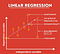# Target Prediction using Single-layer Perceptron and Multilayer Perceptron

This article is mainly written for beginners who want to start their journey into data science or willing to learn Neural Networks from scratch with python coding.

Objective:

• Basic Perceptron

# Basic Perceptron

A perceptron is a neural network unit (an artificial neuron) that does certain computations to detect features or business intelligence in the input data.

Perceptron was introduced by Frank Rosenblatt in 1957. He proposed a Perceptron learning rule based on the original MCP neuron.

A Perceptron is an algorithm for the supervised learning of binary…

# Evaluation Metrics For Regression

## Regression refers to predictive modeling problems that involve predicting a numeric value.Source: Analytics Vidhya

It is different from the classification that involves predicting a class label. Unlike classification, you cannot use classification accuracy to evaluate the predictions made by a regression model.

I have wrote article on evaluation metrics for classification task you can checkout here .

Instead, you must use error metrics specifically designed for evaluating predictions made on regression problems.

In this article, you will discover how to calculate error metrics for regression predictive modeling projects.

# Regression Predictive Modeling:

Predictive modeling is the problem of developing a model using historical data to make a prediction on new data where we do not have the answer.

# Evaluation Metrics for Classification Problem You Should Know with Python Implementation

A classifier is only as good as the metric used to evaluate it.

Evaluating a model is a major part of building an effective machine learning model. The most frequent classification evaluation metric that we use should be ‘Accuracy’. You might believe that the model is good when the accuracy rate is 99%! However, it is not always true and can be misleading in some situations.

When it comes to classification, there are four main types of classification tasks that you may encounter; they are:

• Binary Classification

# Binary Classification

Binary classification refers to those classification tasks…

# All About Activation Functions In Neural Networks

Neural network activation functions are a crucial component of deep learning. Activation functions determine the output of a deep learning model, its accuracy, and also the computational efficiency of training a model — which can make or break a large-scale neural network. Activation functions also have a major effect on the neural network’s ability to converge and the convergence speed, or in some cases, activation functions might prevent neural networks from converging in the first place.

# A Simple Artificial Neuron:

Deep learning models usually consist of many neurons stacked in layers. Let’s consider a single neuron for simplicity.